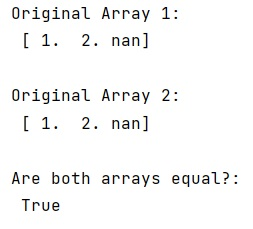# Comparing numpy arrays containing NaN

Learn, how to compare numpy arrays containing NaN in Python?
Submitted by Pranit Sharma, on January 10, 2023

NumPy is an abbreviated form of Numerical Python. It is used for different types of scientific operations in python. Numpy is a vast library in python which is used for almost every kind of scientific or mathematical operation. It is itself an array which is a collection of various methods and functions for processing the arrays.

## NumPy - Comparing arrays containing NaN

While creating a DataFrame or importing a CSV file, there could be some NaN values in the cells. NaN values mean "Not a Number" which generally means that there are some missing values in the cell.

Suppose that we are given two NumPy arrays that contain some nan values and we need to check if these two arrays are equal or not.

For this purpose, the best possible solution is using the == sign (arr1 == arr2). We will use the OR operation where we will define an expression to check whether the array contains nan values or not using the isnan() method.

Let us understand with the help of an example,

## Python code to compare numpy arrays containing NaN

```# Import numpy
import numpy as np

# Creating two arrays
arr1 = np.array([1, 2, np.NaN])
arr2 = np.array([1, 2, np.NaN])

# Display original arrays
print("Original Array 1:\n",arr1,"\n")
print("Original Array 2:\n",arr2,"\n")

# Checking for array equality
res = ((arr1 == arr2) | (np.isnan(arr1) & np.isnan(arr2))).all()

# Display result
print("Are both arrays equal?:\n",res,"\n")
```

Output: